+ +

# Myopia: refraction and axial length

Myopia is an ametropia which is characterized by excessive ocular axial length towards the refractive power of the optics of the eye (horny and crystalline).

Can you quantify the effect of the elongation of the eye on the ocular refraction? What is the effect on the length of the eye the increase in myopia of a diopter? How many diopters of myopia is a millimeter of eye elongation responsible?

To answer these questions, we can make the following schema and perform some simplifications: the myopic eye is by definition such that the retina is combined with the punctum point which is at a finite distance (L) of the master plan previous P.  The image of the object located at the point punctum (noted PR) is formed on the retina, located at a distance the rear main plan P' (the main plans are combined and such that their growth is equal to 1). The source object (located in the punctum point) is in the air (n = 1), and its image form in a medium whose refractive index is that of the glass (no = 1336).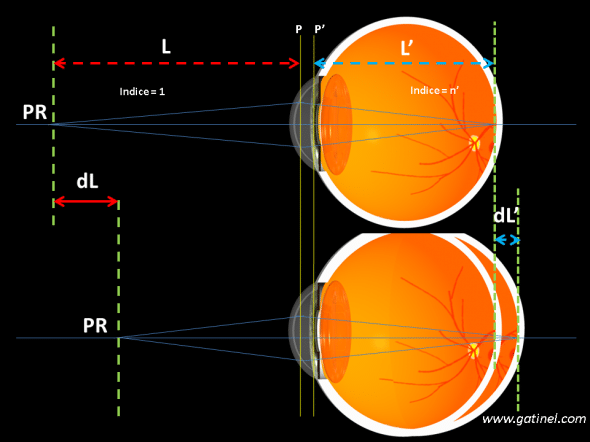Relationship between axial length and myopisation. The diagram represents a myopic eye (top), which increases the axial length (bottom), without changing the other biometric parameters (same cornea, same lens). The increase in the axial length of a dL element' causes a change of the position of the point punctum: the inverse of the distance dL is equal to V0, which is the change from caused vergence (myopisation).

Using the relations of vergences (and being careful to respect the conventions of signs for the expression of distances), we can write the vergence of the eye (V) is equal to: no/l ' + (n/-L).

Let V = no/l '-n/L (1)

The second term in this expression is the vergence of the object, located in the air (n = 1) one can write V0 = 1/L. The expression (1) becomes:

V = no/l '-V0,

Where one gets:

V0 = No/l '-V (2)

Note that for an object at infinity, an Emmetropic eye has an equal to no/l vergence' (V0 equal 0 because L is infinite)

What's happening - you it if we increase the axial length of a dL amount '? This causes a slight variation of the distance of the Punctum point that there is dL, and so the vergence of the dV object0 (= 1/dL).

We're trying to estimate how much varies V0  (which is the change of vergence in the reconciliation of the PR) with dL' (increase the axial length of the eye)?

We have: V = no/l '-V0 where V is a constant related to the characteristics of the concerned eye (the vergence of the entire eye is sum of the respective vergences the cornea and the crystalline lens in practice equal to l). We consider this expression as a function that connects the (variable) and V0

To assess the effect of a small change of the (designated by dL') we can derive V0

compared to dL' in the expression (2) established previously (V0 = No/l '-V)

What gives: dV0 dL'= no /-L' 2

so: dV0 = No dL'/-L' 2

For an Emmetropic eye, the = no /V (because L is at infinity) and we get to the final, by replacing the by don't /V:

dV0 dL =' V2  / No                 (3)

So the change in vergence is equal to the product of the increase of axial length multiplied by the square of the initial eye power divided by no.

Numerical example : If one eye has a vergence of 60 D stretches of 1 mm, what is the induced variation of the vergence of the object (the induced refractive error?):

dV0 = 0.001 x 602 / 1336 = 2.70 D.

So, we can estimate that an increase in the axial length of 1 mm causes a myopia of about-2.75 D, and this is fairly well corroborated what is observed in clinical practice, where it is difficult to establish a strong correlation between axial length and myopic for low myopia (lower to-4 d). These day are related to a "disharmony" between axial length and vergence.

In the light of these data, we can imagine that Nature has chosen another mechanism to allow the eyes to look at net, consisting of a reversible elongation of the eyeball: a lengthening of 1 mm of the eye in effect sufficient to induce a change of vergence of 2.75 D an Emmetropic eye to see net 1/2.75 allowing is 36 cm which is a comfortable reading distance.

You can also apply as a surgical reduction of myopia to "shorten" the eye could use as a rule that myopia is reduced by approximately 2.70 for each mm of reduction of the axial length, D.

In this example, there is a difference of refraction (Anisometropia) between the right eye and the left eye of the same short-sighted patient: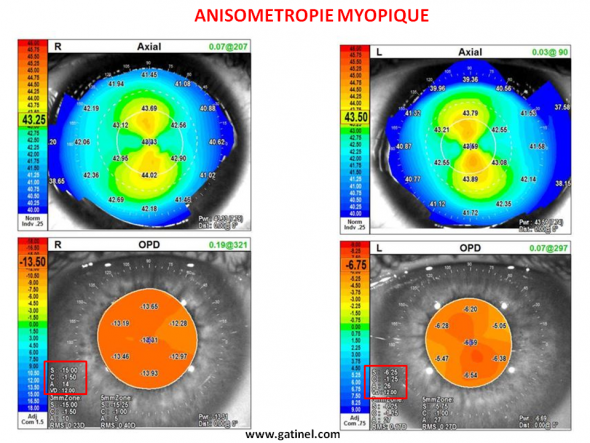Patient aberrometriques (OPD Scan III, Nidek) same topo maps. The right eye (R) presents a high myopia (-15.75 D = spherical equivalent). On the left (L), myopia is much less pronounced (equivalent spherical = - 7 D) corneal topography reveals corneas whose k profile is remarkably similar. The difference in refraction comes almost exclusively from the difference in axial length.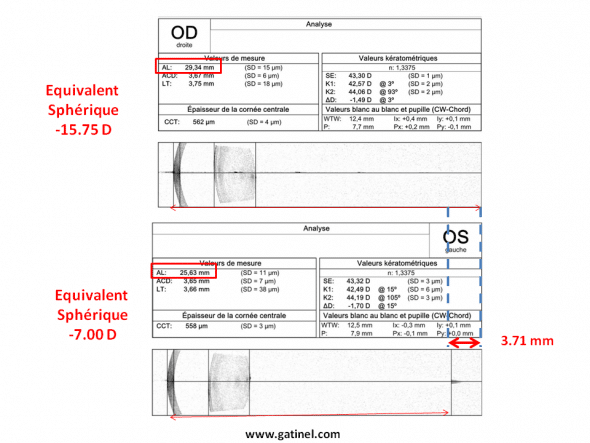The measure of the axial length by interferometric biometry (IOL Master 700, Zeiss) reveals a difference in axial length of 3.71 mm between the eye must (axial length = 29.34 mm) and the left eye (axial length = 25.63 mm).

The following example is borrowed from a different case of Anisometropia (difference in refraction between the two eyes of the same subject). The right eye is Emmetropic (no), and the left eye long-sighted (about + 2.50 D). As predicted by theory, the difference between the respective axial length of the right eye and the left eye is almost equal to 1 mm.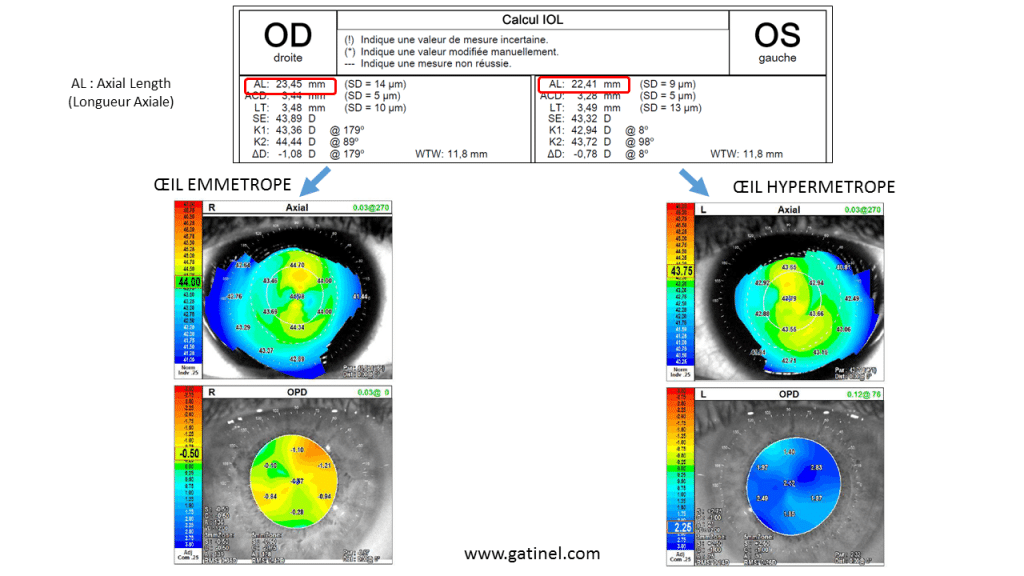The difference in axial length between the right eye and the left eye is close to a millimeter: the difference in refraction (in spherical equivalent) is + 2.50 D. The left eye is farsighted because its axial length is insufficient, and the image formed on the retina is blurred.

In this new example, Central corneal power (vergence), estimated by the keratometry is identical between two eyes that differ significantly in terms of axial length (3 mm).

The first, the longest eye is nearsighted (approximately 6.50 D), and the second, shorter, hypermetropic (about + 3 D). A difference in axial length of 3 mm is as predicted by theory to a difference of close vergence of 10 d.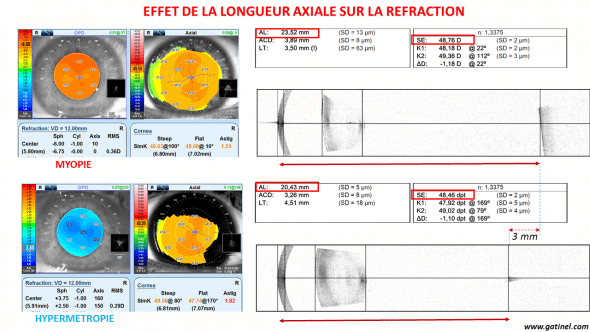The myopic eye, above, shows excessive axial length towards the vergence of the couple "horny + crystalline". In contrast, the hyperopic eye has an insufficient axial length. The eyes have a similar corneal vergence. The effect of the lens (which does not occupy the same position with respect to the cornea in the two case) is probably negligible in view of the significant difference in refraction between the two eyes.

Operations of myopia are classically a corneal reshaping (intended to reduce the corneal vergence of a value equal to that of the magnitude of the myopia). By sculpting the cornea)LASIK or PKR), the laser reduces the thickness; This induces an effective reduction in the length of the eye! However, this reduction is of the order of a few tens of microns (Munnerlyn formula indicates a couple of microns per diopter of myopia treated on an optical zone of 6 mm). For each treated diopter, eye is "shorter" 15 microns;

Using the formula (3), we can estimate the change induced by this shortening of 15 micron to 0.04 D, which is negligible in practice.

The length of the outer segment containing the photosensitive pigment of photoreceptors of the fovea (cones) is approximately 50 microns or 0.000005 meters: that light is focused at the front or at the back of these segments does not in theory their activation.   Using the previous formula (3), you can convert this "spatial tolerance" in 'tolerance of vergence' which is worth...: 0.13 D (either a half quarter diopter). This value is of the same order as the minimum value of vergence changes to which the eye is sensitive!

In clinical practice, there is sometimes a reduction of myopia (or a hypermetropisation) about 1 diopter in case of acute macular edema: the "bulge" of the retinal tissue results in a breakthrough of the plan of the photoreceptors of approximately 200 to 400 microns (therefore a shortening of the length of the eye), which is in line with the refractive change.
Estimate of the diameter of the task of defocus

What is the diameter the task of retinal defocus-induced myopia? This depends on the magnitude of the refractive error and pupillary diameter.

You can rearrange the equation (3) to express the elongation axial (in mm) based on the myopic error (in power)

No dV0 / V2=

V2 / No

dL' =. dV0 No / V2

Either dL' = 0.371 dV0 : 1 diopter of myopia induced 0.371 mm of axial elongation for an eye which the vergence average is 60 d. For such an eye, the effective focal length (LFE) is close to 22 mm, before elongation of a dL value '.

Using the method of identical triangles (see following diagram), you can ask that the diameter of the task of defocus (dB) is equal to: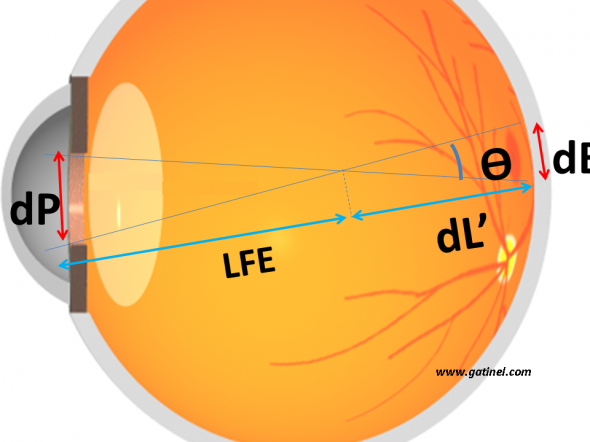The diameter dB of the task of defocus can be estimated from the ratio between the diameter of the entrance pupil, focal length (EFL) effective and dL', the retinal elongation induced by the refractive error

dB = 0,371 x dP x dL'/ 22 or 17 microns for a pupil diameter of 1 mm and 1 diopter error (myopia).